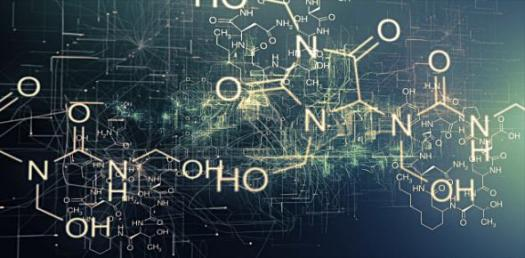# What Do You Know About Chemical Equation? Trivia Quiz

10 Questions | Total Attempts: 105SettingsChemical equation is a symbolic representation of a chemical reaction. A chemical equation also shows us the substances involved in a chemical reaction and energy or catalyst used in the reaction. The elements in an equation are denoted using their symbols. Subscripts are used to indicate the number of atoms of an element present in a chemical reaction. Try this quiz.

• 1.
What is the symbolic representation of chemical reaction?
• A.

Chemical mathematical representation

• B.

Chemical Equation

• C.

Reaction Representation

• D.

Chemical Symbol

• 2.
How is the physical state of chemicals denoted?
• A.

Stating it after the chemical symbol

• B.

Using parentheses

• C.

Stating it before the chemical symbol

• D.

None of these

• 3.
What is the physical state of Mg(s)?
• A.

Solid

• B.

Liquid

• C.

Gas

• D.

Aqueous

• 4.
Which symbol is used on the reaction arrow to denote heat energy?
• A.

~

• B.

• C.

Π

• D.

μ

• 5.
What does the law of conservation of mass dictate?
• A.

No two quantities are thesame

• B.

The quantity of each element does not change in a chemical reaction.

• C.

Masses of each elements changes after chemical reaction

• D.

The quantity of elements varies with it's output quantity after chemical reaction

• 6.
How can a chemical equation be balanced?
• A.

Adding more elements to the equation

• B.

Applying heat

• C.

Changing scaler number

• D.

Reducing elements in excess

• 7.
What's the chemical symbol for hydrochloric acid?
• A.

HyCl

• B.

HyCh

• C.

HCl

• D.

HCLa

• 8.
What is the outcome of the reaction between Hydrochloric acid and Sodium?
• A.

Limestone and water

• B.

Salt and Hydrogen gas

• C.

Salt and water

• D.

Limestone and Hydrogen gas

• 9.
On a chemical equation, where is the reactants written?
• A.

Left hand side

• B.

Right hand side

• C.

Both sides

• D.

On the arrow

• 10.
When an energy is required, where is it denoted?
• A.

Left hand side

• B.

Right hand side

• C.

On the arrow

• D.

None

Related Topics